Name:    4th Grade Operations & Algebraic Thinking - Standard 5 - Practice Test 1

1.

1 6 11 16  21  26  31  ____
What is the next number in this pattern?
 a. 33 c. 40 b. 36 d. 35

2.

21  17  13  9   5  ____
What is the next number in this pattern?
 a. 0 c. 1 b. 3 d. 4

3.

What is the pattern used in this sequence?

22   31  40   49   58
 a. + 9 c. - 10 b. + 10 d. + 8

4.

What is the next shape in this pattern?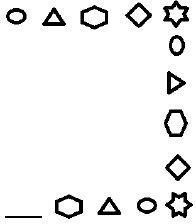a.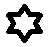c.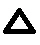b.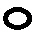d.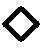5.

What color will appear in space z in this pattern?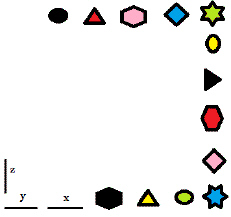a.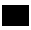c.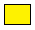b.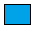d.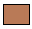6.

Which formula describes the pattern below?

10  11   21  22   32  33
 a. double one c. add 11 b. + 2 d. add 1 + 10

7.

Martha likes to do certain things on certain days.  Look at the chart below.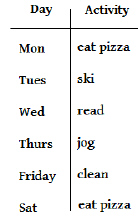According to the chart, what will she do on Sunday?
 a. ski c. rest b. read d. jog

8.

Brett was training for a marathon race.  His training schedule called for him to start the week on Monday with a 6 mile run, then increase it by a pattern of + 3 each day until the end of the week - Sunday.  How far will Brett run on Thursday?
 a. 9 miles c. 6 miles b. 15 miles d. 26 miles

9.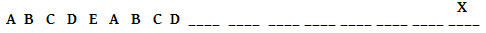a. A c. C b. B d. E

10.

17  21  25  29  33  37
Which description of this pattern is true?
 a. add an even number to an odd number and you get an odd number c. add two numbers and you will get and odd number b. add an odd number to an odd number and you get an odd number d. add two numbers and you will get an even number